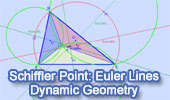# Interactive Schiffler Point: Four Euler Lines. Level: High School, SAT Prep, CollegeThe Euler lines of triangles ABC, AIC, BIC, and AIB respectively, where I is the incenter of ABC, concur at the Schiffler point S.

O, O1, O2, O3 and G, G1, G2, G3 are the circumcenters and centroids of triangles ABC, AIC, BIC, and AIB respectively.

Click the red button () on the figure to start the animation.
Drag points A, C and line AC to change the figure.

Euler Line is the line through the centroid, circumcenter and orthocenter of a triangle.

Centroid is the concurrent point of the medians of a triangle.

Circumcenter is the concurrent point of the perpendicular bisectors of a triangle.

Orthocenter is the concurrent point of the altitudes of a triangle.

Incenter is the concurrent point of the angle bisectors of a triangle.

Concurrent: Two or more lines are said to be concurrent if they intersect at a single point.

Kurt Schiffler (1896-1986), an accomplished amateur geometer, discovered one of the most attractive of the "twentieth-century" triangle centers, now known as the Schiffler point. He introduced the point in the Mathematicorum problem 1018.

Reference: Schiffler, Kurt; Veldkamp, G. R.; van der Spek, W. A. (1985). "Problem 1018". Crux Mathematicorum 11: 51. Solution, vol. 12, pp. 150–152.

Dynamic Geometry: You can alter the figure above dynamically in order to test and prove (or disproved) conjectures and gain mathematical insight that is less readily available with static drawings by hand.

This page uses the TracenPoche dynamic geometry software and requires Adobe Flash player 7 or higher. TracenPoche is a project of Sesamath, an association of French teachers of mathematics.Home | Geometry | Dynamic Geometry | TracenPoche Index | Common Chord | Email